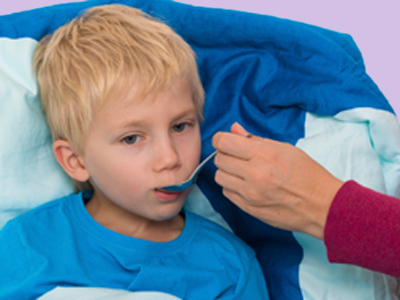Mark has to take 15ml of medicine twice per day for 7 days. He needs 210ml of medicine.

# Solving Problems (Year 5)

In KS2 Maths, we learn to solve problems using addition, subtraction, multiplication, and division. Year Five kids tackle bigger numbers and explore measures like distance, weight, capacity, and time.

Real-life problems mean using addition, subtraction, multiplication, or division. Imagine taking a train from Sheffield to London, changing at Peterborough. The first train is 2 hours, the second is 1 hour. But don't forget the time to change trains and when the second train leaves - your journey might be 4 hours or even more!

Test your skills in this quiz. Can you solve problems with addition, subtraction, multiplication, and division?

1.
6 planks of wood are all 150cm long. We require a continuous run of planks for 8.75m. How much needs chopping off the last plank?
12cm
25cm
50cm
250cm
6 x 150 = 900cm. We only need 8.75m or 875cm so we will need to cut off 25cm
2.
A joint of beef requires 30 minutes per 500g cooking time plus 30 minutes. For how long does a 3.5kg joint of beef require cooking?
1 hour
135 minutes
2 hours
4 hours
If the beef needs 30 minutes per 500g (+30 minutes) then it need 1 hour per kg (+ 30 minutes)
3.
The jug of lemonade contained 2 litres. Gran drank 265ml. How much lemonade was left?
1.735 litres
17.35 litres
173.5 litres
1,735 litres
There are 1,000ml in a litre so to find the answer take 265 away from 2,000, which is 1,735ml or 1.735 litres
4.
Mark has to take 15ml of medicine twice per day for 7 days. How much medicine does he need?
30ml
140ml
105ml
210ml
15ml twice a day is 15 x 2 = 30ml. 30ml over 7 days is 30 x 7 = 210ml
5.
A bag of apples weighs 720g and a bag of pears weigh 645g. How much do the two bags weigh altogether in kg?
1.365kg
13.65kg
136.5kg
1,365kg
720 + 645 = 1,365. There are 1,000g in a kg so the answer is 1.365kg. Did you get the decimal point in the right place?
6.
A box holds 12 eggs. I have 136 eggs. How many boxes do I need to hold all the eggs?
11
12
13
15
136 ÷ 12 = 11 remainder 4 therefore 12 boxes will be required to hold all the eggs even though one box will only contain 4 eggs
7.
Each jacket potato takes 6 minutes to cook in a microwave oven. How many minutes would it take for 9 jacket potatoes to cook?
45 minutes
54 minutes
66 minutes
72 minutes
If you don't know the 9 times table one way to work out 6 x 9 is to multiply 6 by 10 (60) and take away 6 (54)
8.
A box of chocolates has 24 toffees, 49 soft centres and 18 nuts. If I eat 52 chocolates how many are left in the box?
39
41
49
51
24 + 49 + 18 = 91 so I have 91 sweets altogether. 91 - 52 = 39 so if I eat 52 sweets I will have 39 left
9.
I have four ribbons measuring 34mm, 55mm, 87mm and 123mm. What is their total length in cm?
0.299cm
1.99cm
2.9cm
29.9cm
34 + 55 + 87 + 123 = 299. There are 10mm in a cm so to convert mm into cm you divide their number by 10
10.
I have 12 oranges, all of an equal weight, with a total weight of 900g. I eat 5 oranges. What is the total weight of the remaining oranges?
500g
515g
525g
535g
First find the weight of one orange by dividing 900g by 12 which is 75g, so 7 oranges will be 75g x 7 = 525g